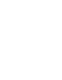Please use this identifier to cite or link to this item: `http://dspace.iua.edu.sd/handle/123456789/3362`
 Title: Interior-Point Methods Applied to Linear and Semi Definite Programming Authors: KADRI HAROUNA ABOUBAKAR LABBO Keywords: المعادلات الخطية Issue Date: 2018 Citation: International University of Africa - Deanship of Graduate Studies Abstract: Most interior-point methods (IPMs) use a modified Newton method to determine the search direction at each iteration. The system of equations corresponding to the modified Newton system can often be reduced to the so-called ''normal equation'' a system of equations whose matrix is positive definite, yet often ill-conditioned. Using an appropriately preconditioned iterative linear solver, the so-called ''the maximum weight basis ( MWB ) preconditioner'' of J. W. O'Neal into infeasible, long-step, primal-dual, path-following algorithms for linear programming ( LP), we show that the number of iterative solver (''inner'') iterations of the algorithms can be uniformly bounded by n ( length of the decision vector) and the condition number of constrains matrix A, while the algorithmic (''outer'') iterations of the IPMs can be polynomially bounded by n and the logarithm of the desired accuracy. We extend our study to semi definite programming in which, basing on the ''Adaptive Preconditioned Steepest Descent'' (APSD) algorithm of J. W. O'Neal, we proposed new algorithm, called ''Adaptive Preconditioned Conjugate Gradient''(APCG). Numerical tests are conducted to compare our algorithm with J.W. O'Neal APSD algorithm basing on execution time. URI: http://dspace.iua.edu.sd/handle/123456789/3362 Appears in Collections: أطروحات الدكتوراه

Files in This Item:
File Description SizeFormat
intro.pdf702.06 kBAdobe PDF